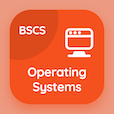Class 6 Online Courses

Grade 6 Math MCQ PDF - Chapters

# Ratio Rate and Speed MCQ Questions Online p. 10

Practice Ratio rate and speed MCQ with answers, ratio rate and speed quiz questions with answers PDF to solve grade 6 math MCQ bank 10 for online classes. Average Speed Multiple Choice Questions and Answers (MCQs), ratio rate and speed quiz questions and answers for online school courses. "Ratio Rate and Speed MCQ" PDF eBook: average speed test prep for online elementary school classes.

"If car travels 40 km/h then the distance car travelled in 50s is" Multiple Choice Questions (MCQ) on ratio rate and speed with choices 300 m, 240 m, 250 m, and 200 m for online school courses. Solve average speed quiz questions for school certificate programs for online certificate courses.

## MCQs on Ratio Rate and Speed

MCQ: If car travels 40 km/h then the distance car travelled in 50s is

240 m
300 m
250 m
200 m